# Let z1=x1+y1i

Let z1=x1+y1i and z2=x2+y2i
Find:
a = Im (z1z2)
b = Re (z1/z2)

Result

a = (Correct answer is: x1*y2+y1*x2-y1*y2)b =  0

#### Solution: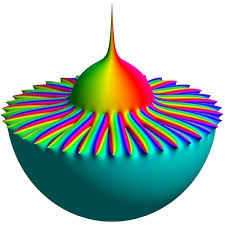We would be pleased if you find an error in the word problem, spelling mistakes, or inaccuracies and send it to us. Thank you!Tips to related online calculators

## Next similar math problems:

• Find x 2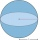Find x, y, and z such that x³+y³+z³=k, for each k from 1 to 100. Write down the number of solutions.
• ProveProve that k1 and k2 are the equations of two circles. Find the equation of the line that passes through the centers of these circles. k1: x2+y2+2x+4y+1=0 k2: x2+y2-8x+6y+9=0
• Functions f,gFind g(1) if g(x) = 3x - x2 Find f(5) if f(x) = x + 1/2
• Mappings of complex numbers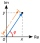Find the images of the following points under mappings: z=3-2j w=2zj+j-1
• Center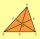In the triangle ABC is point D[1,-2,6], which is the center of the |BC| and point G[8,1,-3], which is the center of gravity of the triangle. Find the coordinates of the vertex A[x,y,z].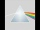Please determine the solvability conditions of the equation, solve the equation and perform the test: x divided by x squared minus 2x plus1 the whole minus x + 3 divided by x squared minus 1 this is equal to 0: x/(x2-2x+1) - (x+3)/( x2-1) = 0
• Expressions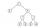Find out value of expressions if a = -1, b =2: x=b - 2a - ab y=a3 - b2 - 2ab z=a2 b3 - a3 b2 w=a + b + a3 - b2
• Curve and lineThe equation of a curve C is y=2x² -8x+9 and the equation of a line L is x+ y=3 (1) Find the x co-ordinates of the points of intersection of L and C. (2) Show that one of these points is also the stationary point of C?Which of the points belong function f:y= 2x2- 3x + 1 : A(-2, 15) B (3,10) C (1,4)
• Sphere from tree pointsEquation of sphere with three point (a,0,0), (0, a,0), (0,0, a) and center lies on plane x+y+z=a
• Intersections 3Find the intersections of the circles x2 + y2 + 6 x - 10 y + 9 = 0 and x2 + y2 + 18 x + 4 y + 21 = 0
• De Moivre's formula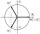There are two distinct complex numbers z such that z3 is equal to 1 and z is not equal 1. Calculate the sum of these two numbers.
• Evaluate expressionIf x=2, y=-5 and z=3 what is the value of x-2y
• My fatherMy father has a big farm. 6/8 of it were planted with mango trees, 1/2 of the remainder are guava trees and the rest 10 trees are santol trees. What is the number of all trees?
• Linear systemSolve a set of two equations of two unknowns: 1.5x+1.2y=0.6 0.8x-0.2y=2
• Sphere equationObtain the equation of sphere its centre on the line 3x+2z=0=4x-5y and passes through the points (0,-2,-4) and (2,-1,1).
• Find the 2Find the term independent of x in the expansion of (4x3+1/2x)8